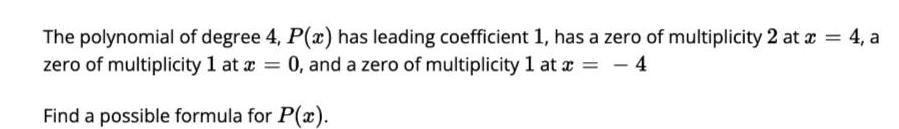Question:

# The polynomial of degree 4, P(x) has leading coefficient 1,

Last updated: 7/27/2022The polynomial of degree 4, P(x) has leading coefficient 1, has a zero of multiplicity 2 at x = 4, a zero of multiplicity 1 at a x=0, and a zero of multiplicity 1 at x = - 4 Find a possible formula for P(x).